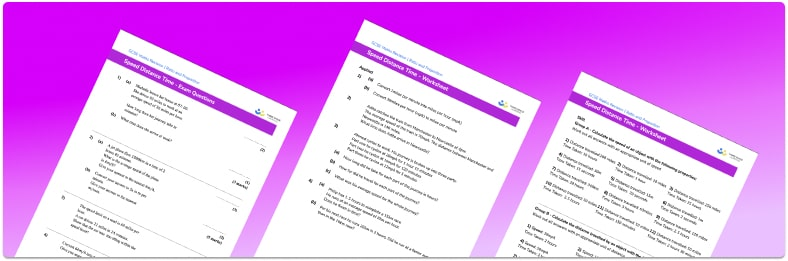# Speed Distance Time Worksheet• Section 1 of the speed distance time worksheet  contains 36 skills-based speed distance time questions, in 3 groups to support differentiation
• Section 2 contains 4 applied speed distance time questions with a mix of worded problems and deeper problem solving questions
• Section 3 contains 4 foundation and higher level GCSE exam style speed distance time questions
• An answer key and a mark scheme for all speed distance time questions are provided
• Questions follow variation theory with plenty of opportunities for students to work independently at their own level
• All questions created by fully qualified expert secondary maths teachers
• Suitable for GCSE maths revision for AQA, OCR and Edexcel exam boards

• This field is for validation purposes and should be left unchanged.

You can unsubscribe at any time (each email we send will contain an easy way to unsubscribe). To find out more about how we use your data, see our privacy policy.

### Speed distance time at a glance

Speed is a compound measure (a measure using more than one quantity), and is calculated as distance ÷ time. This formula calculates the average speed of an object travelling over a known distance for a known time. As it is a compound measure, speed has compound units – the standard metric unit for speed is metres per second, m/s.

We can use the formula for speed to calculate distance by working out speed x time, similarly we can use the formula for speed to calculate time by working out distance divided by speed. In order to manipulate the speed formula it is important that students are able to apply skills from algebra, such as rearranging formulae into different formats.

Distance-time graphs can be used to represent journeys made by objects and these will often relate to real-life examples such as a word problem involving a car journey.

Looking forward, students can then progress to additional ratio and proportion worksheets, for example a or aFor more teaching and learning support on Ratio and Proportion our GCSE maths lessons provide step by step support for all GCSE maths concepts.

## Do you have KS4 students who need more focused attention to succeed at GCSE?There will be students in your class who require individual attention to help them succeed in their maths GCSEs. In a class of 30, it’s not always easy to provide.

Help your students feel confident with exam-style questions and the strategies they’ll need to answer them correctly with our dedicated GCSE maths revision programme.

Lessons are selected to provide support where each student needs it most, and specially-trained GCSE maths tutors adapt the pitch and pace of each lesson. This ensures a personalised revision programme that raises grades and boosts confidence.

Find out more